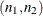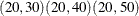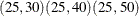#### Grouped-Number-Lists

A grouped-number-list specifies multiple scenarios for numeric values in two or more groups, possibly including missing value indicators to solve for a specific group. The list can assume one of two general forms, a crossed version and a matched version.

##### Crossed Grouped-Number-Lists

The crossed version of a grouped number list consists of a series of number-lists (see the section Number-Lists), one representing each group, with groups separated by a vertical bar (|). The values for each group represent multiple scenarios for that group, and the scenarios for each individual group are crossed to produce the set of all scenarios for the analysis option. For example, you can specify the following six scenarios for the sizesof two groupsas follows:

   GROUPNS = 20 25 | 30 40 50


If the analysis can solve for a value in one group given the other groups, then one of the number-lists in a crossed grouped-number-list can be a missing value indicator (.). For example, in a two-sample t test you can posit three scenarios for the group 2 sample size while solving for the group 1 sample size:

   GROUPNS = . | 30 40 50


Some analyses can involve more than two groups. For example, you can specify 231 = 6 scenarios for the means of three groups in a one-way ANOVA as follows:

   GROUPMEANS = 10 12 | 10 to 20 by 5 | 24

##### Matched Grouped-Number-Lists

The matched version of a grouped number list consists of a series of numeric lists, each enclosed in parentheses. Each list consists of a value for each group and represents a single scenario for the analysis option. Multiple scenarios for the analysis option are represented by multiple lists. For example, you can express the crossed grouped-number-list

   GROUPNS = 20 25 | 30 40 50


alternatively in a matched format:

   GROUPNS = (20 30) (20 40) (20 50) (25 30) (25 40) (25 50)


The matched version is particularly useful when you want to include only a subset of all combinations of individual group values. For example, you might want to pair 20 only with 50, and 25 only with 30 and 40:

   GROUPNS = (20 50) (25 30) (25 40)


If the analysis can solve for a value in one group given the other groups, then you can replace the value for that group with a missing value indicator (.). If used, the missing value indicator must occur in the same group in every scenario. For example, you can solve for the group 1 sample size (as in the section Crossed Grouped-Number-Lists) by using a matched format:

   GROUPNS = (. 30) (. 40) (. 50)


Some analyses can involve more than two groups. For example, you can specify two scenarios for the means of three groups in a one-way ANOVA:

   GROUPMEANS = (15 24 32) (12 25 36)